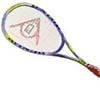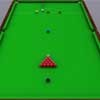#### You may also like### Rain or Shine

Predict future weather using the probability that tomorrow is wet given today is wet and the probability that tomorrow is wet given that today is dry.### Squash

If the score is 8-8 do I have more chance of winning if the winner is the first to reach 9 points or the first to reach 10 points?### Snooker

A player has probability 0.4 of winning a single game. What is his probability of winning a 'best of 15 games' tournament?

# Put Out

##### Age 16 to 18 Challenge Level:

Stage 1. There are 5 yellow balls in bag 1. One ball is transferred to bag 2 which contains an unknown number of green balls.
Stage 2. Bag 2 is then shaken and a ball is selected at random and transferred to bag 1 without seeing its colour.
Stage 3 . Bag 1 is then shaken and a ball is selected at random and transferred to bag 2 without seeing its colour.
Stage 4. Given all this has happened, the probability of selecting a green ball from bag 2 is now 3/5, so how many green balls were there in bag 2 at the outset?

Gordon of Madras College correctly used a tree diagram and the conditional probability of a green ball being chosen given all possible sequences of events leading up to the last draw.

Let ( y 1 , g 1 ) and ( y 2 , g 2 ) denote the numbers of yellow and green balls in the two bags,
e.g. (5, 0) (0, n ) at the outset for bags 1 and 2 respectively.

 Stage 1 A yellow ball is transferred from bag 1 to bag 2. Stage 2 One ball is transferred from bag 2 to bag 1. Stage 3 One ball is transferred from bag 1 to bag 2 There are 3 possible outcomes Probabilities of each outcome Stage 4 A ball is drawn from bag 2. Probability that this ball is green. (5,0) (0, n)pr( y )=1 (4,0) (1, n )pr( y )= 1/( n +1) (5, 0) (0,n )pr( y )=1 (4,0) (1, n ) 1/( n +1) [1/ ( n +1)]* [ n / ( n +1)]pr( g )= n /( n +1) (4, 1) (1, n -1)pr( y )=4/5 (3,1) (2, n -1) 4 n /5( n +1) [4 n / 5( n +1)]* [( n -1)/( n +1)] pr(g) =1/5 (4,0) (1, n ) n /5( n +1) [ n /5( n +1)]* [ n / ( n +1)]

Denote the transfers at stage 2 and 3 by (yellow, yellow), (yellow, green), (green, yellow), (green, green) and note that (yellow, green) is impossible so it has zero probability. At stage 4 a ball is picked at random from bag 2. The probability that this ball (say ball x) is green is given by the sum of the probabilities in the right hand column of the tree diagram above.

pr ( x = green | (yellow, yellow))
 pr ( x = green) = + pr ( x = green | (yellow, green)) + pr ( x = green | (green, yellow)) + pr ( x = green | (green, green))

As we are told pr( x = green) = 3/5
[1 / ( n +1)][ n / ( n +1)] + 0 + [4 n / 5( n +1)][( n - 1) / ( n +1)] + [ n /5( n +1)][ n / ( n +1)] = 3/5.

Multiplying by 5( n +1) 2

5 n + 4 n ( n - 1) + n 2 = 3( n + 1) 2

2 n 2 - 5 n - 3 = 0

( n - 3)(2 n + 1) = 0.

So there were 3 green balls in bag 2 at the start (discounting the impossible solution n = - $\frac {1}{2}$).